#### Implementation in VisualApplets

In Fig. 355 you can see the basic design structure of the VA design for the calculation of the HOG feature. For a grayscale image from a camera in CameraLink base configuration the Histogram of Oriented Gradients is calculated in the HierarchicalBox HOG. This histogram is sent to PC via DMA in the design "HOG_9Bins_Histogram.va". In the VA designs "HOG_4Bins_HistogramMax.va" and "HOG_9Bins_HistogramMax.va" the maximum component is forwarded to PC. In the following we explain the design structure of "HOG_4Bins_HistogramMax.va". It is analog for the other two designs. We explain possible differences in the designs for the corresponding issues. The default platform is marathon VCL. The design implementations can easily adapted to other platforms or camera configurations.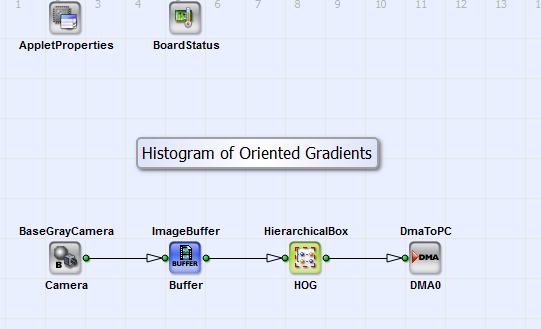Figure 355. Basic Design structure of the VA designs "HOG_9Bins_Histogram.va","HOG_9Bins_HistogramMax.va" and "HOG_4Bins_HistogramMax.va"

You can see the content of the HierarchicalBox HOG in Fig. 356.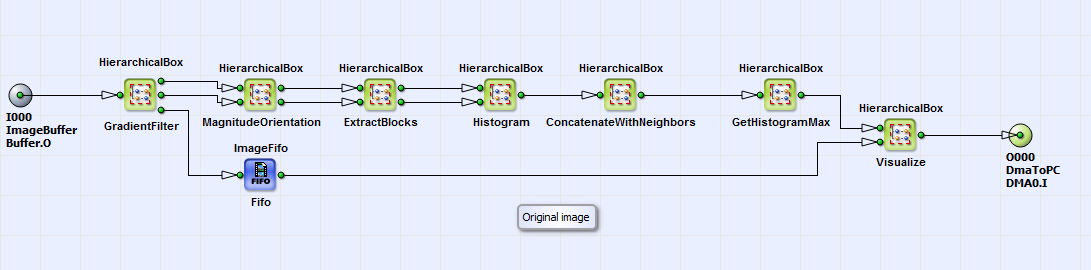Figure 356. Content of HierarchicalBiox HOG

For the original input image the gradients in x- and y- direction are calculated in the HierarchicalBox GradientFilter. The implementation is shown in Fig. 47. The operator FIRkernelNxM generates a 3x3 kernel. The operators FIRoperatorNxM_VerticalEdges and FIRoperatorNxM_HorizontalEdges select according to eq. 47 the gradients in x- and y-direction.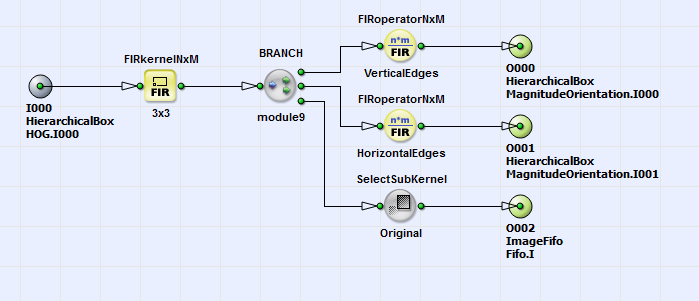Figure 357. Content of HierarchicalBox GradientFilter

For this gradients the magnitude and orientation according to eq. 48 is implemented in the box MagnitudeOrientation (content and structure see Fig. 358).Detailed comments in the box help to understand the implemented formula.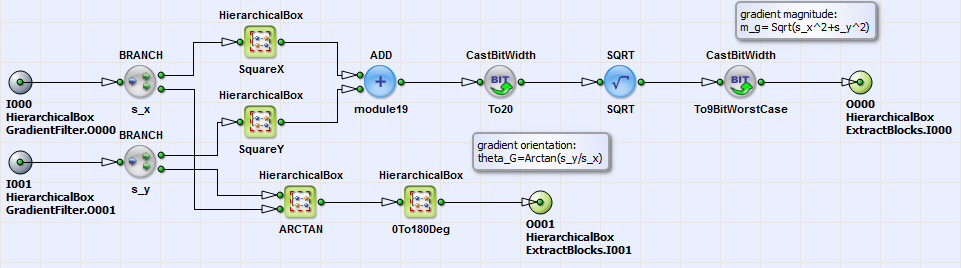Figure 358. Content of HierarchicalBox MagnitudeOrientation

The grouping of the pixels in cells of 8x8 pixels is performed in the HierarchicalBox ExtractBlocks. The output links of this box forward the gradient magnitude and orientation of the cells in form of lines of size 64 pixels. This information is input for the calculation of the Histogram of Oriented Gradients, which is performed in the HierarchicalBox Histogram. You can see its content in Fig. 359.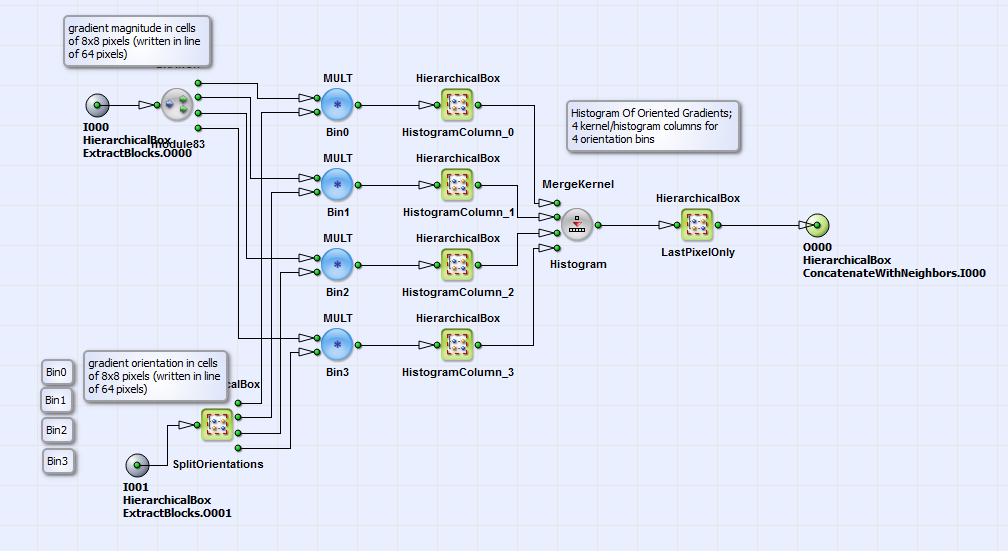Figure 359. Content of HierarchicalBox Histogram

Here an assignment of the gradient orientations, which can be in a region of to , to four bins is performed in the box SplitOrientations. The bins are , , and . For the designs "HOG_9Bins_Histogram.va" and "HOG_9Bins_HistogramMax.va" nine bins ( to in steps of ) exist. The assignment of the gradient orientation to a certain bin is done the way, that a weight of this orientation for the bin is calculated. As an example the weighting and therefor assignment to bin 1, which is , is shown in Fig. 360.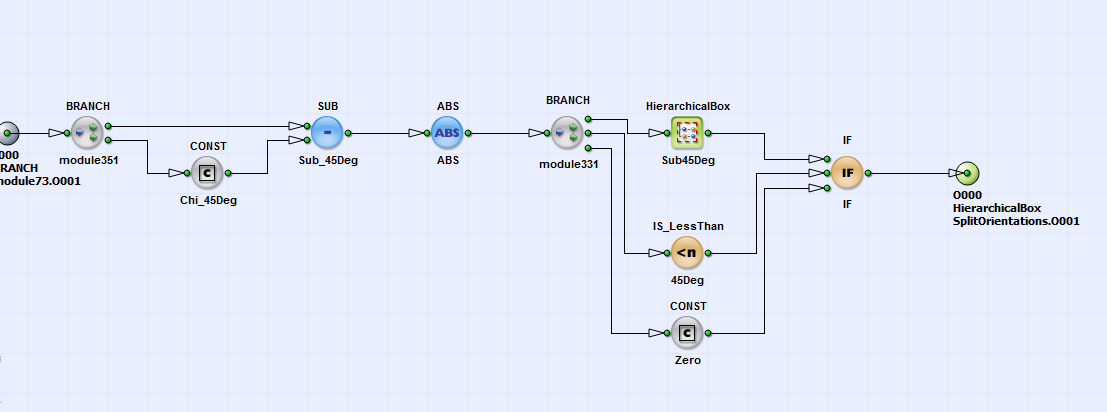Figure 360. Content of HierarchicalBox Bin1

Here the weight according to eq. 49 is calculated for bin 1. The angle distance between the bins is in this example (operator CONST_Phi_45Deg in box Sub45Deg). The angle bin orientation for bin 1 is also (operator CONST_Chi_45Deg). The weighting factor for each bin is then multiplied with the gradient magnitudes (see Fig. 359). In the boxes HistogramColumn_0 to HistogramColumn_3 the content of each histogram column (for each bin) according to eq. 50 is calculated as the sum of the weighted gradient magnitudes over all image pixels. The Histogram of Oriented Gradients is then generated with merging the histogram columns with the operator MergeKernel_Histogram. Only the last pixel of each kernel component is the true value of the histogram columns, so it is selected in the box LastPixelOnly. To account for local gradient changes the Histogram of Oriented Gradients for each cell is combined to blocks of size 2x2 cells per block with an overlap of (see theory part of this section). The combination of the cells to blocks is performed in the box ConcatenateWithNeighbors. See its content in Fig. 361.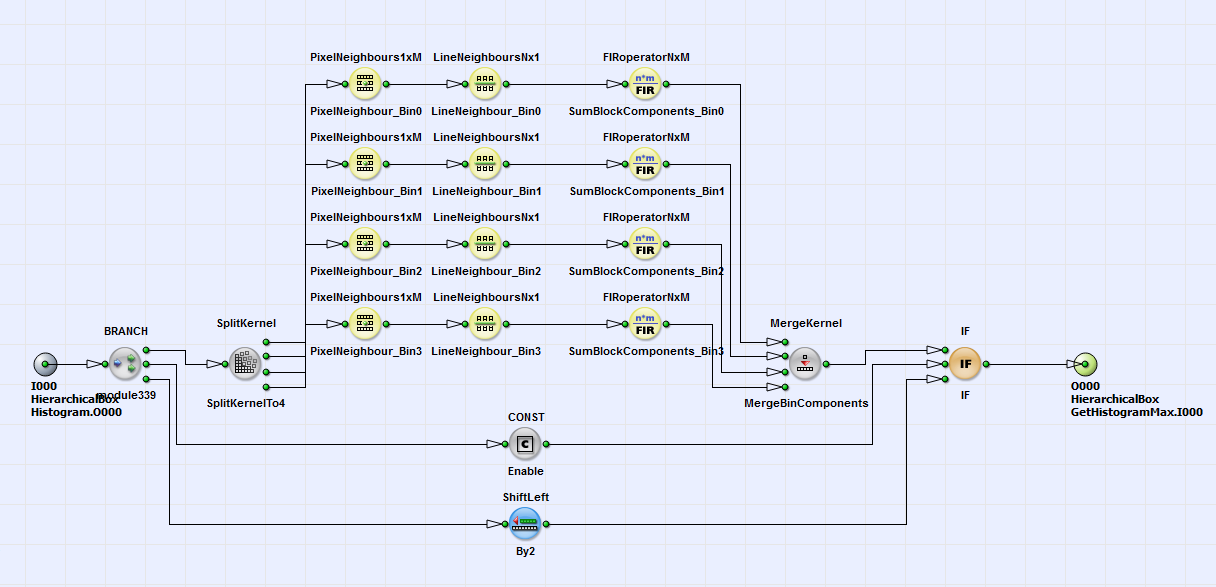Figure 361. Content of HierarchicalBox ConcatenateWithNeighbors

For each cell (represented by a single pixel) with information about the Histogram of Oriented Gradients, the histogram columns for each bin are separated with the operator SplitKernel. For each kernel component the next cell neighbors in x and y- direction are calculated with the operators PixelNeighbours1xM and LineNeighboursNx1.The operator FIRoperatorNxM sums the information of the 2x2 cells up. No normalization is performed here. As an alternative implementation the grouping of the cells to blocks can be omitted by setting the operator Const_Enable to zero. The maximum of the calculated Histogram of Oriented Gradients is selected in box GetHistogramMax (see Fig. 356). Its content is shown in Fig. 362. In the design "HOG_9Bins_Histogram.va" the selection of the histogram maximum is omitted.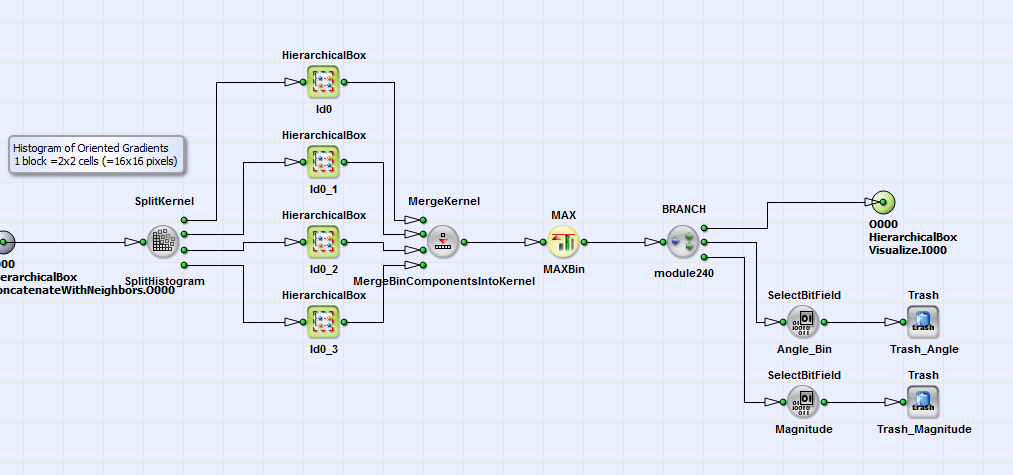Figure 362. Content of HierarchicalBox GetHistogramMax

Finally the maximum of the histogram or the histogram itself is visualized in box Visualize (see Fig 356) as red lines. In this box (path: box Draw, EnableOriginal) you have the possibility to visualize the original image for a number of frames together with the HOG feature by setting the parameters ModuloCount_Period (counts frames), Is_InRange (set frame range) and Const_Enable or always (setting Const_Enable to one). As last step the HOG feature is transferred to PC via the operator DmaToPC_DMA0. Here the HOG feature can be used as descriptor for object detection in Support Vector Machines (SVP) or also Convolutional Neural Networks (CNN).